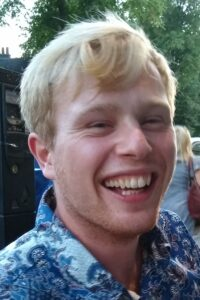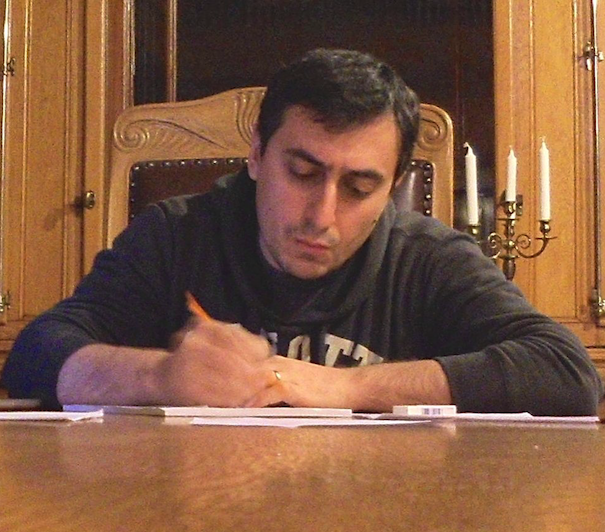# PeoplePieter Blue is interested in the analysis of partial differential equations (PDEs). I’m interested in hyperbolic PDEs arising from mathematical physics, and most of my research has been on models for stability of black holes as a solution of the Einstein equation. I have generally used tools that are applicable to nonlinear equations to derive estimates for solutions of linear equations, and I’m looking to extend this to nonlinear problems.Nikolaos Bournoveas is interested in Nonlinear hyperbolic and kinetic differential equations.Tony Carbery studies classical harmonic analysis and related areas. More recently it has become clear that there are deep combinatorial and geometrical issues that underpin many of the problems of modern harmonic analysis. Typically, these combinatorial manifestations are easy to state but not so easy to resolve. Much of Carbery’s recent research activity has been concerned with trying to highlight and push forward this perspective.Martin Dindos is interested in Elliptic and Parabolic equations, particularly the solvability of boundary value problems (Dirichlet, Neumann, Regularity) and with rough coefficients on domains with limited regularity.Jonathan Hickman is interested in Euclidean harmonic analysis, in particular questions related to continuum incidence geometry. Examples include the study of the Kakeya problem, Fourier restriction/extension operators and geometric maximal functions.Aram Karakhanyan studies Monge-Ampere Equations, Nonlinear elasticity, and Free boundaries.Linhan Li is interested in the interplay of harmonic analysis, partial differential equations, and geometric measure theory. Examples include the solvability of boundary value problems for elliptic equations with minimal regularity assumptions on the coefficients and/or on the boundary of the domain in question, and characterizations of geometric properties of sets in terms of properties of solutions of a partial differential equation.Hiro Oh studies Nonlinear Partial Differential Equations and Harmonic Analysis. In particular, he studies (deterministic and stochastic) nonlinear dispersive Hamiltonian PDEs, using the techniques from PDEs, nonlinear Fourier analysis, and probability. Mainly, short and long time behavior of solutions such as well-posedness (existence, uniqueness, and stability of solutions) in both deterministic and probabilistic settings, existence of invariant measures and their properties, solitons, growth of higher Sobolev norms related to weak turbulence, etc.Jan Sbierski is interested in mathematical general relativity, hyperbolic partial differential equations, and Lorentzian geometry. A large part of his research is concerned with the formation of singularities inside black holes and their geometric structure, as well as with the problem of global uniqueness of the time-evolution under the Einstein equations, which is also known as the strong cosmic censorship conjecture.Leonardo Tolomeo  is interested in stochastic PDEs, dispersive equations and harmonic analysis. In particular, he studies the effect that randomness has on the behaviour of the solutions of nonlinear (dispersive) PDEs, either because of the presence of a random forcing, or in order to study the statistical behaviour of the flow. This endeavour mixes techniques from nonlinear PDEs, harmonic analysis and probability.Jim Wright studies Euclidean harmonic analysis, oscillatory integrals, generalised singular and maximal Radon transforms, and stability/ uniformity issues related to these objects. Recently he has also been interested in discrete analogues of these problems in settings where harmonic analysis interacts nicely with number theory.

# PhD Students